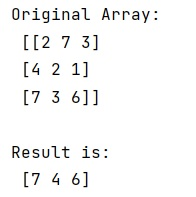# NumPy selecting specific column index per row by using a list of indexes

Given a NumPy array, we have to select specific column index per row by using a list of indexes.
Submitted by Pranit Sharma, on December 27, 2022

NumPy is an abbreviated form of Numerical Python. It is used for different types of scientific operations in python. Numpy is a vast library in python which is used for almost every kind of scientific or mathematical operation. It is itself an array which is a collection of various methods and functions for processing the arrays.

## Selecting specific column index per row by using a list of indexes

Suppose we are given a two-dimensional NumPy array with some rows and columns. We need to select some specific column based on the index which is itself an element of the list given to use.

For this purpose, we will use np.arrange method() which will take a total length of the array and the index list.

Let us understand with the help of an example,

## Python code to select specific column index per row by using a list of indexes

```# Import numpy
import numpy as np

# Creating a numpy array
arr = np.array([[2, 7, 3],[4, 2, 1],[7, 3, 6]])

# Display original array
print("Original Array:\n",arr,"\n")

# Creating a list of indices
lst = [1,0,2]

# using arrange method
res = arr[np.arange(len(arr)), lst]

# Display result
print("Result is:\n",res,"\n")
```

Output: